### Home > CCA > Chapter 10 > Lesson 10.2.4 > Problem10-69

10-69.
1. Factor each of the following expressions completely. Be sure to look for any common factors. Homework Help ✎

1. 4x2 − 12x

2. 3y2 + 6y + 3

3. 2m3 + 7m2 + 3m

4. 3x2 + 4x − 4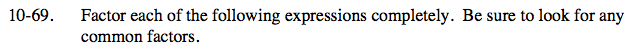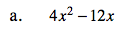There is a common factor of 4x.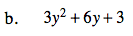There is a common factor of 3.

Now factor the quadratic.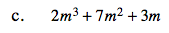There is a common factor of m.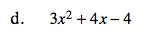Use a diamond and generic rectangle to factor.